# Excel date functions with formula examples

This is the final part of our Excel Date Tutorial that offers an overview of all Excel date functions, explains their basic uses and provides lots of formula examples.

Microsoft Excel provides a ton of functions to work with dates and times. Each function performs a simple operation and by combining several functions within one formula you can solve more complex and challenging tasks.

In the previous 12 parts of our Excel dates tutorial, we have studied the main Excel date functions in detail. In this final part, we are going to summarize the gained knowledge and provide links to a variety the formula examples to help you find the function best suited for calculating your dates.

## Excel DATE function

`DATE(year, month, day)` returns a serial number of a date based on the year, month and day values that you specify.

When it comes to working with dates in Excel, DATE is the most essential function to understand. The point is that other Excel date functions not always can recognize dates entered in the text format. So, when performing date calculations in Excel, you'd better supply dates using the DATE function to ensure the correct results.

Here are a few Excel DATE formula examples:

`=DATE(2015, 5, 20)` - returns a serial number corresponding to 20-May-2015.

`=DATE(YEAR(TODAY()), MONTH(TODAY()), 1)` - returns the first day of the current year and month.

`=DATE(2015, 5, 20)-5` - subtracts 5 days from May 20, 2015.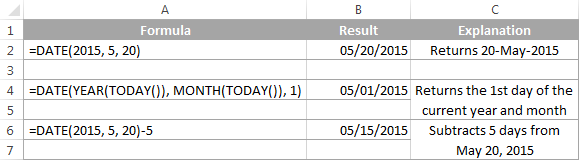At first sight, the Excel DATE function looks very simple, however, it does have a number of specificities pointed out in the Excel DATE tutorial.

Below you will find a few more examples where the Excel DATE function is part of bigger formulas:

## Excel TODAY function

The `TODAY()` function returns today's date, exactly as its name suggests.

TODAY is arguably one of the easiest Excel functions to use because it has no arguments at all. Whenever you need to get today's date in Excel, enter the following formula is a cell:

`=TODAY()`

Apart from this obvious use, the Excel TODAY function can be part of more complex formulas and calculations based on today's date. For example, to add 7 days to the current date, enter the following formula in a cell:

`=TODAY()+7`

To add 30 weekdays to today's date excluding weekend days, use this one:

`=WORKDAY(TODAY(), 30)`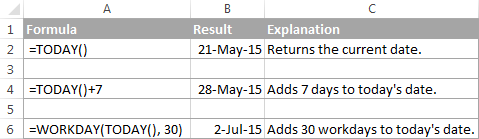Note. The date returned by the TODAY function in Excel updates automatically when your worksheet is recalculated to reflect the current date.

For more formula examples demonstrating the use of the TODAY function in Excel, please check out the following tutorials:

## Excel NOW function

`NOW()` function returns the current date and time. As well as TODAY, it does not have any arguments. If you wish to display today's date and current time in your worksheet, simply put the following formula in a cell:

`=NOW()`

Note. As well as TODAY, Excel NOW is a volatile function that refreshes the returned value every time the worksheet is recalculated. Please note, the cell with the NOW() formula does not auto update in real-time, only when the workbook is reopened or the worksheet is recalculated. To force the spreadsheet to recalculate, and consequently get your NOW formula to update its value, press either Shift+F9 to recalculate only the active worksheet or F9 to recalculate all open workbooks.

For more details, please see How to use NOW function in Excel.

## Excel DATEVALUE function

`DATEVALUE(date_text)` converts a date in the text format to a serial number that represents a date.

The DATEVALUE function understands plenty of date formats as well as references to cells that contain "text dates". DATEVALUE comes in really handy to calculate, filter or sort dates stored as text and convert such "text dates" to the Date format.

A few simple DATEVALUE formula examples follow below:

`=DATEVALUE("20-may-2015")`

`=DATEVALUE("5/20/2015")`

`=DATEVALUE("may 20, 2015")`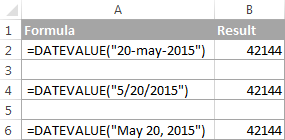And the following examples demonstrate how the DATEVALUE function can help with solving real-life tasks:

## Excel TEXT function

In the pure sense, the TEXT function cannot be classified as one of Excel date functions because it can convert any numeric value, not only dates, to a text string.

With the TEXT(value, format_text) function, you can change the dates to text strings in a variety of formats, as demonstrated in the following screenshot.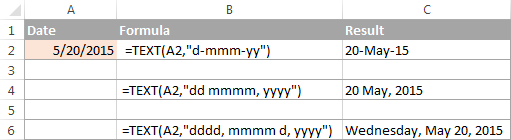Note. Though the values returned by the TEXT function may look like usual Excel dates, they are text values in nature and therefore cannot be used in other formulas and calculations.

Here are a few more TEXT formula examples that you may find helpful:

## Excel DAY function

`DAY(serial_number)` function returns a day of the month as an integer from 1 to 31.

Serial_number is the date corresponding to the day you are trying to get. It can be a cell reference, a date entered by using the DATE function, or returned by other formulas.

Here are a few formula examples:

`=DAY(A2)` - returns the day of the month from a date in A2

`=DAY(DATE(2015,1,1))` - returns the day of 1-Jan-2015

`=DAY(TODAY())` - returns the day of today's date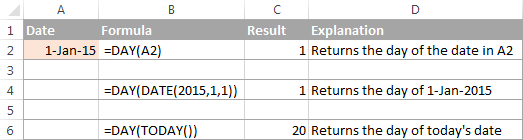## Excel MONTH function

`MONTH(serial_number)` function in Excel returns the month of a specified date as an integer ranging from 1 (January) to 12 (December).

For example:

`=MONTH(A2)` - returns the month of a date in cell A2.

`=MONTH(TODAY())` - returns the current month.

The MONTH function is rarely used in Excel date formulas on its own. Most often you would utilize it in conjunction with other functions as demonstrated in the following examples:

For the detail explanation of the MONTH function's syntax and plenty more formula examples, please check out the following tutorial: Using the MONTH function in Excel.

## Excel YEAR function

`YEAR(serial_number)` returns a year corresponding to a given date, as a number from 1900 to 9999.

The Excel YEAR function is very straightforward and you will hardly run into any difficulties when using it in your date calculations:

`=YEAR(A2)` - returns the year of a date in cell A2.

`=YEAR("20-May-2015")` - returns the year of the specified date.

`=YEAR(DATE(2015,5,20))` - a more reliable method to get the year of a given date.

`=YEAR(TODAY())` - returns the current year.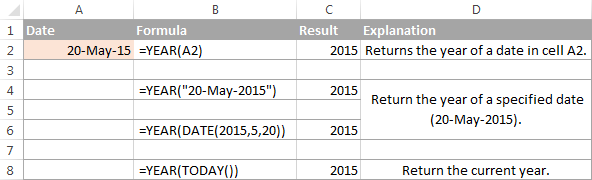## Excel EOMONTH function

`EOMONTH(start_date, months)` function returns the last day of the month a given number of months from the start date.

Like most of Excel date functions, EOMONTH can operate on dates input as cell references, entered by using the DATE function, or results of other formulas.

A positive value in the `months` argument adds the corresponding number of months to the start date, for example:

`=EOMONTH(A2, 3)` - returns the last day of the month, 3 months after the date in cell A2.

A negative value in the months argument subtracts the corresponding number of months from the start date:

`=EOMONTH(A2, -3)` - returns the last day of the month, 3 months before the date in cell A2.

A zero in the months argument forces the EOMONTH function to return the last day of the start date's month:

`=EOMONTH(DATE(2015,4,15), 0)` - returns the last day in April, 2015.

To get the last day of the current month, enter the TODAY function in the start_date argument and 0 in months:

`=EOMONTH(TODAY(), 0)`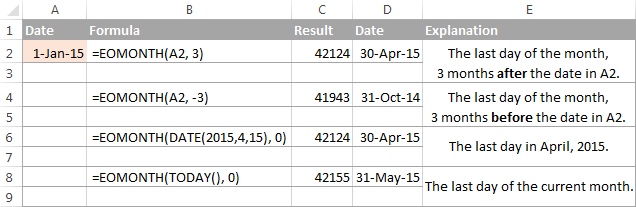You can find a few more EOMONTH formula examples in the following articles:

## Excel WEEKDAY function

`WEEKDAY(serial_number,[return_type])` function returns the day of the week corresponding to a date, as a number from 1 (Sunday) to 7 (Saturday).

• Serial_number can be a date, a reference to a cell containing a date, or a date returned by some other Excel function.
• Return_type (optional) - is a number that determines which day of the week shall be considered the first day.

You can find the complete list of available return types in the following tutorial: Day of the week function in Excel.

And here are a few WEEKEND formula examples:

`=WEEKDAY(A2)` - returns the day of the week corresponding to a date in cell A2; the 1st day of the week is Sunday (default).

`=WEEKDAY(A2, 2)` - returns the day of the week corresponding to a date in cell A2; the week begins on Monday.

`=WEEKDAY(TODAY())` - returns a number corresponding to today's day of the week; the week begins on Sunday.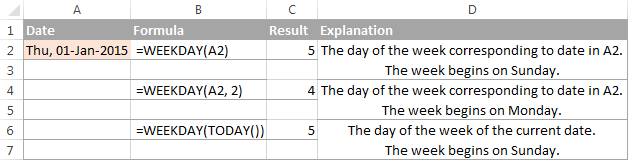The WEEKDAY function can help you determine which dates in your Excel sheet are working days and which ones are weekend days, and also sort, filter or highlight workdays and weekends:

## Excel DATEDIF function

`DATEDIF(start_date, end_date, unit)` function is specially designed to calculate the difference between two dates in days, months or years.

Which time interval to use for calculating the date difference depends on the letter you enter in the last argument:

`=DATEDIF(A2, TODAY(), "d")` - calculates the number of days between the date in A2 and today's date.

`=DATEDIF(A2, A5, "m")` - returns the number of complete months between the dates in A2 and B2.

`=DATEDIF(A2, A5, "y")` - returns the number of complete years between the dates in A2 and B2.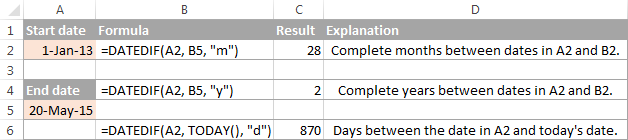These are just the basic applications of the DATEDIF function and it is capable of much more, as demonstrated in the following examples:

## Excel WEEKNUM function

`WEEKNUM(serial_number, [return_type])` - returns the week number of a specific date as an integer from 1 to 53.

For example, the below formula returns 1 because the week containing January 1 is the first week in the year.

`=WEEKNUM("1-Jan-2015")`

The following tutorial explains all the specificities on the Excel WEEKNUM function: WEEKNUM function - calculating week number in Excel.

Alternatively you can skip directly to one of the formula examples:

## Excel EDATE function

`EDATE(start_date, months)` function returns the serial number of the date that is the specified number of months before or after the start date.

For example:

`=EDATE(A2, 5)` - adds 5 months to the date in cell A2.

`=EDATE(TODAY(), -5)` - subtracts 5 months from today's date.

For a detailed explanation of EDATE formulas illustrated with formula examples, please see: How to use EDATE function in Excel.

## Excel YEARFRAC function

`YEARFRAC(start_date, end_date, [basis])` function calculates the proportion of the year between 2 dates.

This very specific function can be used to solve practical tasks such as calculating age from date of birth.

## Excel WORKDAY function

`WORKDAY(start_date, days, [holidays])` function returns a date N workdays before or after the start date. It automatically excludes weekend days from calculations as well as any holidays that you specify.

This function is very helpful for calculating milestones and other important events based on the standard working calendar.

For example, the following formula adds 45 weekdays to the start date in cell A2, ignoring holidays in cells B2:B8:

`=WORKDAY(A2, 45, B2:B85)`

For the detailed explanation of WORKDAY's syntax and more formula examples, please check out:

## Excel WORKDAY.INTL function

`WORKDAY.INTL(start_date, days, [weekend], [holidays])` is a more powerful variation of the WORKDAY function introduced in Excel 2010.

WORKDAY.INTL allows calculating a date N number of workdays in the future or in the past with custom weekend parameters.

For example, to get a date 20 workdays after the start date in cell A2, with Monday and Sunday counted as weekend days, you can use either of the following formulas:

`=WORKDAY.INTL(A2, 20, 2, 7)`

or

`=WORKDAY.INTL(A2, 20, "1000001")`

Of course, it might be difficult to grasp the essence from this short explanation, but more formula examples illustrated with screenshots will make things really easy:

## Excel NETWORKDAYS function

`NETWORKDAYS(start_date, end_date, [holidays])` function returns the number of weekdays between two dates that you specify. It automatically excludes weekend days and, optionally, the holidays.

For example, the following formula calculates the number of whole workdays between the start date in A2 and end date in B2, ignoring Saturdays and Sundays and excluding holidays in cells C2:C5:

`=NETWORKDAYS(A2, B2, C2:C5)`

You can find a comprehensive explanation of the NETWORKDAYS function's arguments illustrated with formula examples and screenshots in the following tutorial:

## Excel NETWORKDAYS.INTL function

`NETWORKDAYS.INTL(start_date, end_date, [weekend], [holidays])` is a more powerful modification of the NETWORKDAYS function available in Excel 2010 and later. It also returns the number of weekdays between two dates, but lets you specify which days should be counted as weekends.

Here is a basic NETWORKDAYS formula:

`=NETWORKDAYS(A2, B2, 2, C2:C5)`

The formula calculates the number of workdays between the date in A2 (start_date) and the date in B2 (end_date), excluding the weekend days Sunday and Monday (number 2 in the weekend parameter), and ignoring holidays in cells C2:C5.

Hopefully, this 10K foot view on the Excel date functions has helped you gain the general understanding of how date formulas work in Excel. If you want to learn more, I encourage you to check out the formula examples referenced on this page. I thank you for reading and hope to see you again on our blog next week!

## You may also be interested in

1. Dear Team,
I have a list of dates over a 5 year period, and would like to be able to add a column to show what day each date was, so I can filter data by day.
Apart from using filter, and chosing every 7 days for 5 years, is there any other way of doing this?

Thank you for any help

2. Aloha, I would like to create a formula that calculates the difference between dates that also stops calculating once action is completed.

I am calculating the amount of time it takes to process transactions, but I don't know how make the date stop counting once the action is complete. Any assistance is greatly appreciated.

• Hello!
To prevent your date from automatically changing, you can use several methods:
1. Use Shortcuts to insert the current date and time